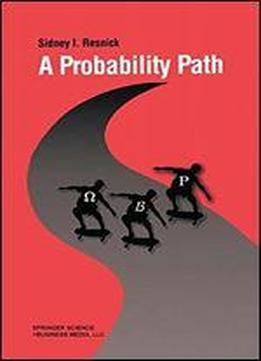# A Probability Path by Sidney Resnick / 2003 / English / PDF

Many probability books are written by mathematicians and have the built in bias that the reader is assumed to be a mathematician coming to the material for its beauty. This textbook is geared towards beginning graduate students from a variety of disciplines whose primary focus is not necessarily mathematics for its own sake. Instead, A Probability Path is designed for those requiring a deep understanding of advanced probability for their research in statistics, applied probability, biology, operations research, mathematical finance, and engineering.

views: 466